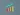# What Is A Histogram?You’ve likely heard of histograms but may find yourself wondering—what is a histogram?

A histogram is a graph that shows how often data is found in a certain range. The bars on a histogram are usually shown in descending order, with the most frequent data at the top.

The histogram will have a bar for each range, and the height of the bar will show how often the data is found in that range. The bars will be ordered from the smallest to the largest value.

## Is A Histogram The Same Thing As A Bar Chart?Histogram vs bar graph working processes is pretty different. A histogram and a bar chart are both specific types of graphs, but they are used for different purposes. For example, a histogram is used to show the distribution of data, while a bar chart is used to compare data.

In contrast to a histogram, a bar chart is a graph that is used to compare data. It is made up of bars that show the different values of data. The bars are usually shown in order from smallest to largest, and the length of the bar represents the value of the data.

## What Are The Uses For A Histogram?There are many uses for histograms. One use is to help you understand your data. Histograms can help you identify the shape of the distribution, which can give you clues about the nature of the data. For example, if the data is symmetrical, then it is likely that the data is from a normal distribution.

How to read a histogram? Let’s see what types of data you are going to see in the Histograms. Histograms can also be used to compare two or more sets of data. By looking at the histograms, you can see how the distributions of the data sets compare. This can be helpful when you are trying to decide whether or not the data sets are from the same distribution.

Another use for histograms is to help you find values that are unusual. By identifying the values that are at the tails of the distribution, you can find the data values that are most unusual. This can be helpful when you are trying to find out which data values are most important.

Finally, histograms can be used to make predictions. By looking at the shape of the distribution, you can often make guesses about the values that will fall in the middle of the distribution. This can be helpful when you are trying to make decisions about the future.

## What Are The Different Types Of Histograms?Many of the users keep asking how to create a histogram in excel. Yes, you can create the histogram model in excel. Only you have to install the analysis toolpak and excel add-in for creating the graph.

Here are the three types of histograms.

### 1. Frequency Histogram

The most common type of histogram is the frequency histogram. This histogram is used to show how often different values occur in a data set. This histogram is a graph that shows how the data is distributed.

###### Canva – User Reviews, Pros & Cons | Graphic Designing Software

The x-axis of the histogram shows the different values in the data set, and the y-axis shows the frequency of each value.

### 2. Relative Frequency Histogram

Another type of histogram is the relative frequency histogram. This histogram is used to show how the data is distributed relative to the maximum value in the data set.

The relative frequency histogram is similar to the frequency histogram, but the y-axis shows the relative frequency of each value. This histogram is useful when you have a data set with a large maximum value and you want to see how the data is distributed relative to that value.

### 3. Cumulative Frequency Histogram

The last type of histogram is the cumulative frequency histogram. This histogram is used to show how the data is distributed cumulatively from the minimum value in the data set.

The cumulative frequency histogram is similar to the frequency histogram, but the y-axis shows the cumulative frequency of each value. This histogram is useful when you have a data set with a significant minimum value and you want to see how the information is distributed cumulatively from that value.

## Conclusion:

Histograms are one of the most popular and effective ways to visualize data. They are used to display the distribution of data and to help identify any patterns or outliers. Overall, a histogram is a fantastic tool for data visualization. So now you know the histogram and regular bar graphs are not the same. You can create the graph in the Excel front. What is your opinion? Share your ideas in the comment sections.